Get instant live expert help with Excel or Google Sheets“My Excelchat expert helped me in less than 20 minutes, saving me what would have been 5 hours of work!”

#### Post your problem and you'll get expert help in seconds

Your message must be at least 40 characters
Our professional experts are available now. Your privacy is guaranteed.

# Convert date to month and year

We can convert a date to month and year using two simple formulas. We will use the TEXT, MONTH and YEAR functions to perform this conversion. In the first example, we will use the TEXT function and in the second; we will use the MONTH and YEAR functions.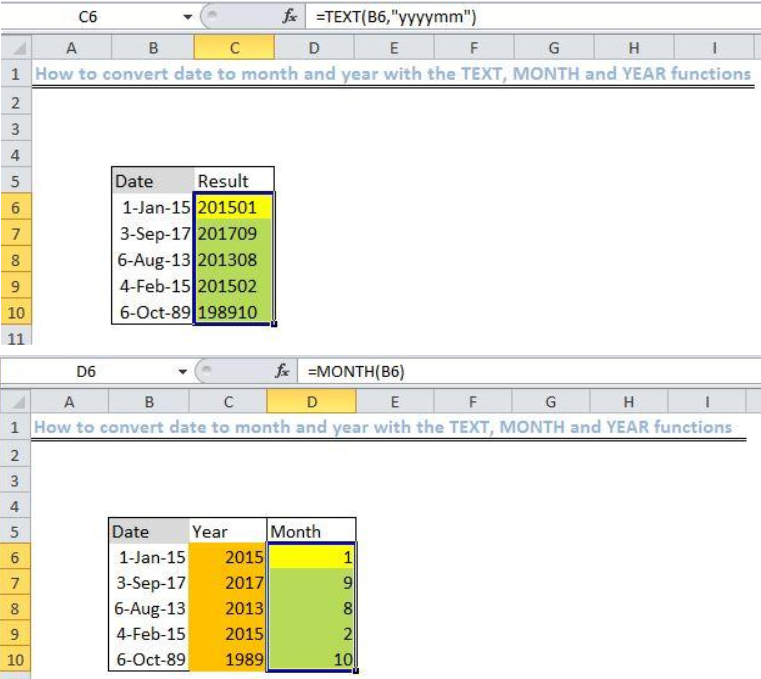Figure 1: Result of convert date to month and year using the TEXT function

## General formula

`=TEXT(date,“yyyymm”)`

## Formula

`=TEXT(B6,"yyyymm")`

## Setting up the Data

• We will set up the data by inputting hypothetical dates in Column B
• Column C is where we will input the formula to arrive at the result.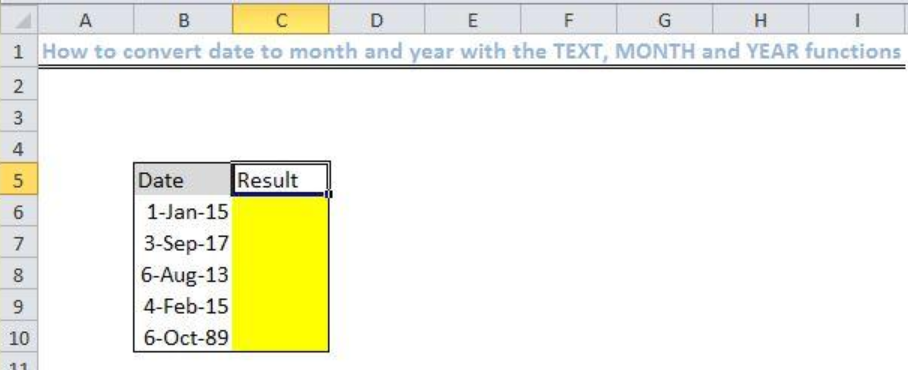Figure 2: Setting up the Data

## Convert date to a month and year with TEXT (B6)

• We will place our cursor in Cell C6
• We will insert the formula below into the Cell C6
`=TEXT(B6,"yyyymm")`
• We will press the enter keyFigure 3: Applying the TEXT function

• We will click on Cell C6 again
• We will double click on the fill handle tool (a little plus sign found at the bottom right of Cell C6) and drag down the formula to the other cells.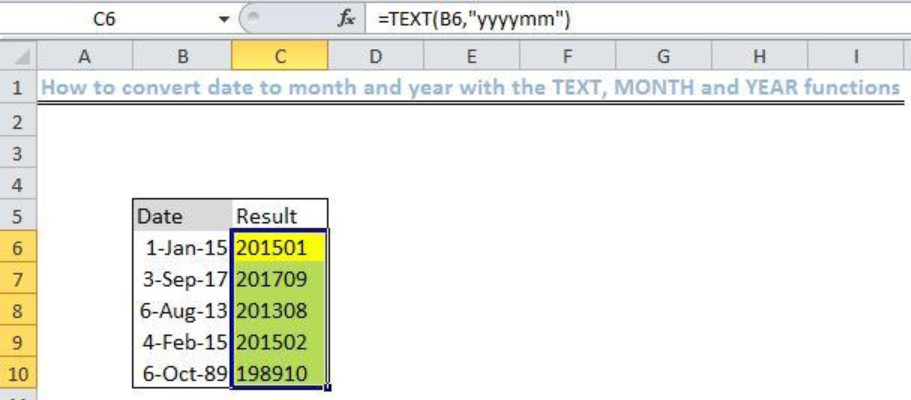Figure 4: Result of using the TEXT function to convert date

## An alternative; convert date to month and year using the Month and Year functions

Step 1: Converting date to year using YEAR function

• We will find the year and month for our set of hypothetical dates
• Columns C and D will contain our year and month results
• In Cell C6, we will enter the formula below and press the enter key
`=YEAR(B6)`
• Again we use the fill handle tool to drag down the formula to other cells in the given column.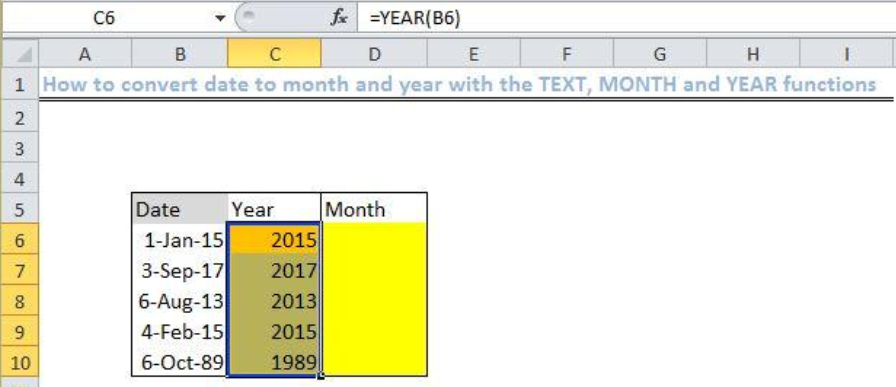Figure 5: Result of using the YEAR function to convert date

Step 2: Converting date to month using MONTH function

• In Cell D6, we will enter the formula below
`=MONTH(B6)`
• We will press the enter key.
• Using the fill handle, we will copy the formula to other cells in the specified column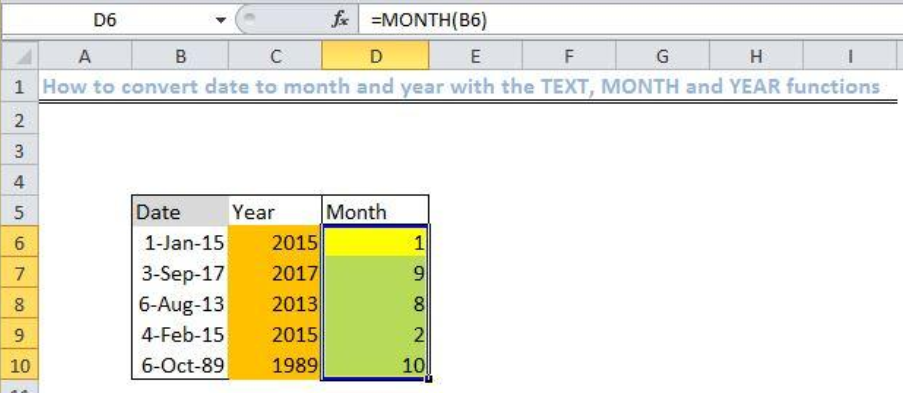Figure 6: Result of using the Month function to convert date

## Explanation

`=TEXT(B6,"yyyymm")`

• The TEXT function works on the number format specified and returns with a result in the form of text.

`=YEAR(B6)`

• The Excel YEAR function will extract the year from the date in the cell and return with the Year as a 4-digit value.

`=MONTH(B6)`

• We use the Excel MONTH function to extract the month from the specified date and return with the date of the month.

## Note

• The Date must be a valid Excel date (serial numbers that begin with 1).
• If Date is not valid, then the functions will return with a #VALUE error.

## Instant Connection to an Expert through our Excelchat Service

Most of the time, the problem you will need to solve will be more complex than a simple application of a formula or function. If you want to save hours of research and frustration, try our live Excelchat service! Our Excel Experts are available 24/7 to answer any Excel question you may have. We guarantee a connection within 30 seconds and a customized solution within 20 minutes.

### Did this post not answer your question? Get a solution from connecting with the expert.Another blog reader asked this question today on Excelchat:## Subscribe to Excelchat.coAnother blog reader asked this question today on Excelchat: